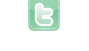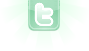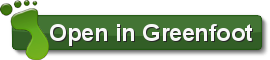Report as inappropriate.

# Maze Beta

This is a maze program. To start it you need to arrow through the menu to select your
difficutly. Once yor difficulty is selected you will see a maze. The object of the
game is to move your dot from the starting position to the orange-ish section. You
move the dot using the arrow keys, and it leaves a red line so you can see where you

This program is still in a beta version so there may be bugs. It would be appreciated
if you told me if you found a bug.

12/11 Update
Dot scrolling error fixed, you move left and right instead of diagonally.
Dot still can't move through the maze vertically.

12/12 Update
Tried Builderboy2005's solution, it almost works. The object can move through
the world but will only scroll some of the time. Why won't it always scroll?

12/13 Update
Figured out a few mistakes, and nearly fixed the scrolling engine. One glitch is
that the scrolling on the x axis only happeens if you reach all the way to the edge.
What am I doing wrong?
Versions, and descriptions filled out

12/14 Update
Tried lirki's solution and it works like a charm. Still can't figure out why viewport

12/15 Update
Finshed Menu now has an easy, medium, hard, and insane level.
shakes.

12/19 Update
I created a victory scene, also you now can win my scenario, finally, I finished my
sound support, so now the sound successfully loops. All that is left to do to release
maze version 1.0 is to comeplete the documentations. If everything works out, it will
be ready by the end of the week.

12/20
Fixed bug so sound now plays online. Each dificulty now has 4 differrent version, so
you won't always have the same maze when you select a difficulty.

NOTICE: Maze version 1.0 has been released. Click on the website link to view it.

5475 views / 518 in the last 7 days

7 votes | 0 in the last 7 daysThere is no HTML 5 translation of this scenario available.
View legacy version (requires Java plugin)

This scenarios uses Java features that are not available for use after conversion to HTML 5. Please try the legacy version, which requires the Java plugin to be installed.

Why isn't the sound playing? It works perfectly as a jar file and in greenfoot.
A new version of this scenario was uploaded on Sun Dec 20 03:11:39 UTC 2009
because of java applet security, you can't access your midi file like you normally do. instead, you have to use some java.net stuff. I coded this b4, so here you go, just replace the play code with this. //you have to import these. put it at the top of the code. import java.net.URL; import java.io.IOException; /** * Play a Midi file. */ public static void playMidiFile(String fileName) { try { URL url = getClass().getClassLoader().getResource(fileName); if(url == null) throw new IOException("File not found: " + fileName); //file does not exist Sequence sequence = MidiSystem.getSequence(url); sequencer.open(); sequencer.setSequence(sequence); sequencer.start(); } catch(Exception ex) { ex.printStackTrace(); } }
A new version of this scenario was uploaded on Sun Dec 20 15:19:49 UTC 2009
A new version of this scenario was uploaded on Sun Dec 20 15:35:30 UTC 2009
A new version of this scenario was uploaded on Sun Dec 20 17:05:48 UTC 2009
A new version of this scenario was uploaded on Sun Dec 20 19:26:49 UTC 2009
A new version of this scenario was uploaded on Sun Dec 20 19:32:45 UTC 2009
A new version of this scenario was uploaded on Sun Dec 20 19:55:23 UTC 2009
I COMPLETED INSANE! :) :) :) :) :) :) :) :) :) :) :) :) :) :) :) :) :) :) :) :) :) :) :) :) :) :) :) :) :) :) :) :) :) :) :) :) :) :) :) :) :) :) :) :) :) :) :) :) :) :) :) :) :) :) :) :) :) :) :) :) :) :) :) :) :) :) :) :) :) :) :) :) :) :) :) :) :) :) :) :) :) :) :) :) :) :) :) :) :) :) :) :) :) :) :) :) :) :) :) :) :) :) :) :) :) :) :) :) :) :) :) :) :) :) :) :) :) :) :) :) :) :) :) :) :) :) :) :) :) :) :) :) :) :) :) :) :) :) :) :) :) :) :) :) :) :) :) :) :) :) :) :) :) :) :) :) :) :) :) :) :) :) :) :) :) :) :) :) :) :) :) :) :) :) :) :) :) :) :) :) :) :) :) :) :) :) :) :) :) :) :) :) :) :) :) :) :) :) :) :) :) :) :) :) :) :) :) :) :) :) :) :) :) :) :) :) :) :) :) :) :) :) :) :) :) :) :) :) :) :) :) :) :) :) :) :) :) :) :) :) :) :) :) :) :) :) :) :) :) :) :) :) :) :) :) :) :) :) :) :) :) :) :) :) :) :) :) :) :) :) :) :) :) :) :) :) :) :) :) :) :) :) :) :) :) :) :) :) :) :) :) :) :) :) :) :) :) :) :) :) :) :) :) :) :) :) :) :) :) :) :) :) :) :) :) :) :) :) :) :) :) :) :) :) :) :) :) :) :) :) :) :) :) :) :) :) :) :) :) :) :) :) :) :) :) :) :) :) :) :) :) :) :) :) :) :) :) :) :) :) :) :) :) :) :) :) :) :) :) :) :) :) :) :) :) :) :) :) :) :) :) :) :) :) :) :) :) :) :) :) :) :) :) :) :) :) :) :) :) :) :) :) :) :) :) :) :) :) :) :) :) :) :) :) :) :) :) :) :) :) :) :) :) :) :) :) :) :) :) :) :) :) :) :) :) :) :) :) :) :) :) :) :) :) :) :) :) :) :) :) :) :) :) :) :) :) :) :) :) :) :) :) :) :) :) :) :) :) :) :) :) :) :) :) :) :) :) :) :) :) :) :) :) :) :) :) :) :) :) :) :) :) :) :) :) :) :) :) :) :) :) :) :) :) :) :) :) :) :) :) :) :) :) :) :) :) :) :) :) :) :) :) :) :) :) :) :) :) :) :) :) :) :) :) :) :) :) :) :) :) :) :) :) :) :) :) :) :) :) :) :) :) :) :) :) :) :) :) :) :) :) :) :) :) :) :) :) :) :) :) :) :) :) :) :) :) :) :) :) :) :) :) :) :) :) :) :) :) :) :) :) :) :) :) :) :) :) :) :) :) :) :) :) :) :) :) :) :) :) :) :) :) :) :) :) :) :) :) :) :) :) :) :) :) :) :) :) :) :) :) :) :) :) :) :) :) :) :) :) :) :) :) :) :) :) :) :) :) :) :) :) :) :) :) :) :) :) :) :) :) :) :) :) :) :) :) :) :) :) :) :) :) :) :) :) :) :) :) :) :) :) :) :) :) :) :) :) :) :) :) :) :) :) :) :) :) :) :) :) :) :) :) :) :) :) :) :) :) :) :) :) :) :) :) :) :) :) :) :) :) :) :) :) :) :) :) :) :) :) :) :) :) :) :) :) :) :) :) :) :) :) :) :) :) :) :) :) :) :) :) :) :) :) :) :) :) :) :) :) :) :) :) :) :) :) :) :) :) :) :) :) :) :) :) :) :) :) :) :) :) :) :) :) :) :) :) :) :) :) :) :) :) :) :) :) :) :) :) :) :) :) :) :) :)